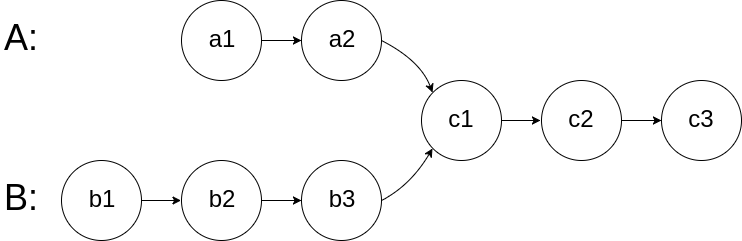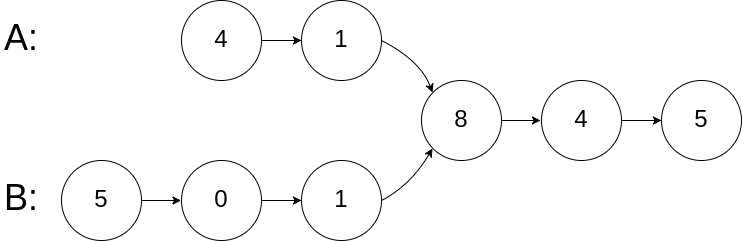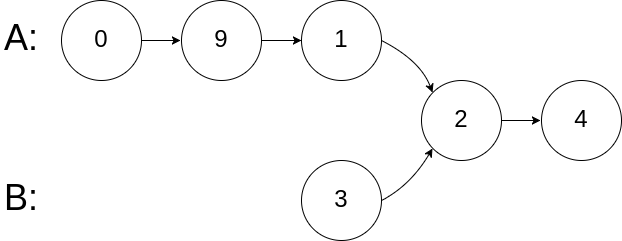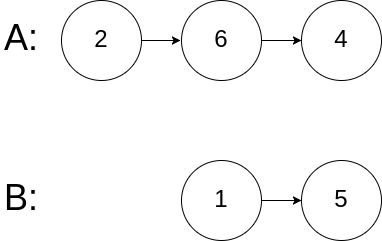# 160. Intersection of Two Linked Lists

## Description

Write a program to find the node at which the intersection of two singly linked lists begins.

For example, the following two linked lists:begin to intersect at node c1.

Example 1:``````Input: intersectVal = 8, listA = [4,1,8,4,5], listB = [5,0,1,8,4,5], skipA = 2, skipB = 3
Output: Reference of the node with value = 8
Input Explanation: The intersected node's value is 8 (note that this must not be 0 if the two lists intersect). From the head of A, it reads as [4,1,8,4,5]. From the head of B, it reads as [5,0,1,8,4,5]. There are 2 nodes before the intersected node in A; There are 3 nodes before the intersected node in B.
``````

Example 2:``````Input: intersectVal = 2, listA = [0,9,1,2,4], listB = [3,2,4], skipA = 3, skipB = 1
Output: Reference of the node with value = 2
Input Explanation: The intersected node's value is 2 (note that this must not be 0 if the two lists intersect). From the head of A, it reads as [0,9,1,2,4]. From the head of B, it reads as [3,2,4]. There are 3 nodes before the intersected node in A; There are 1 node before the intersected node in B.
``````

Example 3:``````Input: intersectVal = 0, listA = [2,6,4], listB = [1,5], skipA = 3, skipB = 2
Output: null
Input Explanation: From the head of A, it reads as [2,6,4]. From the head of B, it reads as [1,5]. Since the two lists do not intersect, intersectVal must be 0, while skipA and skipB can be arbitrary values.
Explanation: The two lists do not intersect, so return null.
``````

Tags: Math, String

## 题意

• 如果两个链表不相交，则返回 null；
• 在函数结束时，两个链表必须保持原来的结构；
• 链表中不存在环；
• 你的代码需要的时间复杂度是 O(n)O(n)，额外的空间复杂度是 O(1)O(1)；

## 题解

### 思路1

。。。。

``````

``````

```